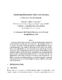## Constructing Deterministic Finite-State Automata in Recurrent Neural Networks##### Files
CS-TR-3460.ps(893.63 KB)
Recurrent neural networks that are {\it trained} to behave like deterministic finite-state automata (DFAs) can show deteriorating performance when tested on long strings. This deteriorating performance can be attributed to the instability of the internal representation of the learned DFA states. The use of a sigmoidal discriminant function together with the recurrent structure contribute to this instability. We prove that a simple algorithm can {\it construct} second-order recurrent neural networks with a sparse interconnection topology and sigmoidal discriminant function such that the internal DFA state representations are stable, i.e. the constructed network correctly classifies strings of {\it arbitrary length}. The algorithm is based on encoding strengths of weights directly into the neural network. We derive a relationship between the weight strength and the number of DFA states for robust string classification. For a DFA with $n$ states and $m$ input alphabet symbols, the constructive algorithm generates a programmed" neural network with $O(n)$ neurons and $O(mn)$ weights. We compare our algorithm to other methods proposed in the literature. Revised in February 1996 (Also cross-referenced as UMIACS-TR-95-50)